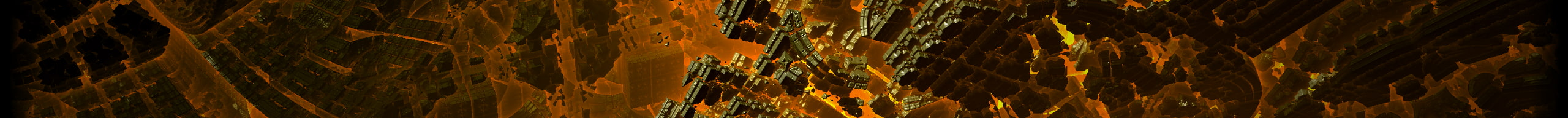# 29a.chby Jonas Wagner# Hand drawn lines using javascript canvas

By

For an upcoming game I'm working on I want the lines to look hand drawn. I found a nice paper on the subject. My algorithm is inspired by that paper, but not an exact implementation. But the results look good anyway. I also wrote a method to draw circles, but it needs improvement. Please let me know if you use it anywhere. :)

``````
function fuzz(x, f){
return x + Math.random()*f - f/2;
}

// estimate the movement of the arm
// x0: start
// x1: end
// t: step from 0 to 1
function handDrawMovement(x0, x1, t){
return x0 + (x0-x1)*(
15*Math.pow(t, 4) -
6*Math.pow(t, 5) -
10*Math.pow(t,3)
)
}

// hand draw a circle
// ctx: Context2D
// x, y: Coordinates
function handDrawCircle(ctx, x, y, r){
var steps = Math.ceil(Math.sqrt(r)*3);

var f = 0.12*r;

// distortion of the circle
var xs = 1.0+Math.random()*0.1-0.05;
var ys = 2.0-xs;

ctx.moveTo(x+r*xs, y);

for(var i = 1; i <= steps; i++)
{
var t0 = (Math.PI*2/steps)*(i-1);
var t1 = (Math.PI*2/steps)*i;
var x0 = x+Math.cos(t0)*r*xs;
var y0 = y+Math.sin(t0)*r*ys;
var x1 = x+Math.cos(t1)*r*xs;
var y1 = y+Math.sin(t1)*r*ys;

ctx.quadraticCurveTo(fuzz(x0, f), fuzz(y0, f), x1, y1);
ctx.moveTo(x1, y1);
}
}

// inspired by this paper
// http://iwi.eldoc.ub.rug.nl/FILES/root/2008/ProcCAGVIMeraj/2008ProcCAGVIMeraj.pdf
function handDrawLine(ctx, x0, y0, x1, y1){
ctx.moveTo(x0, y0)

var d = Math.sqrt((x1-x0)*(x1-x0)+(y1-y0)*(y1-y0))

var steps = d/25;
if(steps < 4) {
steps = 4;
}

// fuzzyness
var f = 8.0;
for(var i = 1; i <= steps; i++)
{
var t1 = i/steps;
var t0 = t1-1/steps
var xt0 = handDrawMovement(x0, x1, t0)
var yt0 = handDrawMovement(y0, y1, t0)
var xt1 = handDrawMovement(x0, x1, t1)
var yt1 = handDrawMovement(y0, y1, t1)
ctx.quadraticCurveTo(fuzz(xt0, f), fuzz(yt0, f), xt1, yt1)
ctx.moveTo(xt1, yt1)
}
}
``````